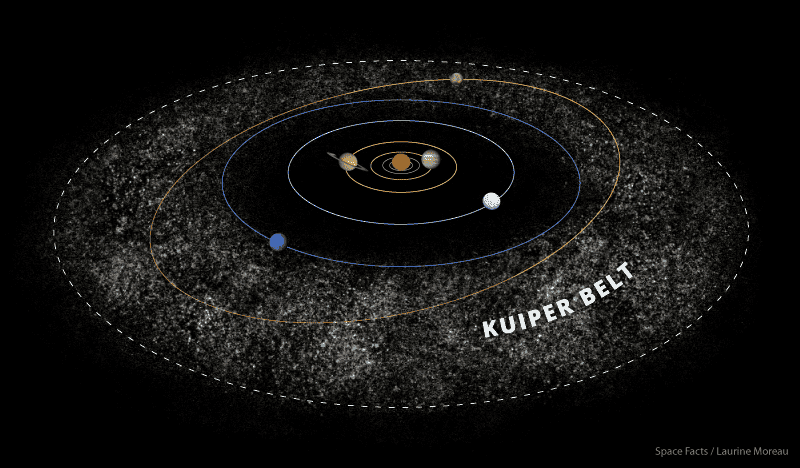What is the distance between the objects in the Kuiper belt? Is the density of objects high and the probability of a spacecraft colliding with objects in this area?

Keith_McClary
I read that if you lived on an asteroid in the Asteroid Belt, you would never see another asteroid (contrary to movie depictions). I think Kuiper is much less dense.

I read that if you lived on an asteroid in the Asteroid Belt, you would never see another asteroid (contrary to movie depictions). I think Kuiper is much less dense.
According to this image, the density of the Kuiper belt is much higher than that of the asteroid belt
My question is, what is the average distance of objects in the Kuiper belt?The above is a drawing. It is not an accurate representation, as it exaggerates the scales for illustrative purposes. On a to-scale picture one wouldn't see anything - no asteroids, no planets - just black space with a bright dot in the middle.

Here's how the densities of the two belts can be roughly compared, using commonly accessible data:

Let's assume the distribution to be doughnut-shaped, which it roughly is. Most asteroids lie between 2 and 3 AU, at inclinations lower than 15 degrees. We can approximate it as a torus at the distance of 2.5 AU, with 0.5 AU thickness (I.e. R and r, respectively).
The mass in the KB is mostly concentrated between 30 and 50 AU, at inclinations of less than 10 degrees. Let's assume a torus at R=40 AU with r=10 AU thickness.

Comparing the two volumes, we find out that the KB occupies roughly 6.7 thousand times more space than the AB.

At the same time, the mass contained within the AB is roughly 4% of the mass of the Moon, which is about 5 thousandth of the mass of the Earth. The mass in the KB is estimated as 2% of Earth mass. Which means the KB is about 4 times the mass of the AB.

I.e. there is four times the mass, within almost seven thousand times the volume. The density in the Kuiper Belt is then some 1.75 thousand times lower than that of the Asteroid Belt - and that was already low in absolute terms. To hit anything with a spacecraft one would need careful aiming, planned months or years ahead. Or colossaly bad luck.

••davenn, berkeman and Bystander
Keith_McClary
KB is about 4 times the mass of the AB.
Wikipedia says:
The Kuiper belt ... is similar to the asteroid belt, but is far larger – 20 times as wide and 20–200 times as massive.

This is the KB mass estimate I used: https://arxiv.org/abs/1810.09771
The mass of the asteroid belt is well established.

Note 1, that even if we allowed for two orders of magnitude higher mass, it'd still mean the KB being significantly less dense than the AB.
Note 2, the Scattered Disc is not included in the aforementioned mass estimate. It's perfectly possible that there is many times more mass hiding out there, including a large planet. But, since the SD extends to ~1000AU, including it would only drive the average density down.

••davenn and Keith_McClary